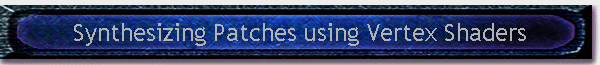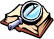#Written by Robert Dunlop Microsoft DirectX MVP< Previous: Page 1 Page 2 Next: Page 3 >

## Streamlining the calculations

However, the processing overhead can be greatly reduced with a bit of consideration.  The first thing that we can do is cutout two thirds of the operations right off the bat.  While complex surfaces would usually employ splines that interpolate vertex coordinates as the parameter, since we are using a uniform grid the X and Z of each control point will be implicit.  Our splines will only need to control a 1-D parameter, the altitude.

To further optimize the calculation, we will look at ways that we can pre-calculate as much as possible.  If we look back on Equation 1, you will see that the spline calculation contains a scalar constant as well as a constant basis matrix.  By pre-multiplying these by the control points to form a 4D vector, the calculation for a a point on a spline segment can be reduced to a single dot product operation:

q(t) = (1,t,t2,t3) • (P2, (P3-P1)/2, (2P1-5P2+4P3-P4)/2, (-P1+3P2-3P3+P4)/2)

Equation 4

Since we will be calculating multiple values from each spline segment, we can pre-calculate the second term of this equation as a 4D vector for each segment, using a function like this:

 ```void preCalcCatmullRom(D3DXVECTOR4 *v,float p1,float p2,float p3,float p4) { v->x=p2; v->y=(p3-p1)*0.5f; v->z=p1-2.5f*p2+2.0f*p3-0.5f*p4; v->w=-0.5f*p1+1.5f*p2-1.5f*p3+0.5f*p4; }``` Listing 1
 P1  P2  P3  P4 * Basis Matrix * 0.5 -> P'1  P'2   P'3  P'4

When calculating a point in a patch, we have to perform this operation with four parallel spline segments.  To bundle these operations together, we can place the four 4D vectors that will be used for each spline calculation into a 4x4 matrix:

 Note: Assumes existence of a float array splines[NUM_SPLINES][NUM_SPLINES] that contains the height at the control points. ```D3DXMATRIX splineMat; D3DXVECTOR4 *pMatV=(D3DXVECTOR4 *) &splineMat;``````for (int i=0;i<4;i++) { preCalcCatmullRom(pMatV, height[x+i][z], height[x+i][z+1], height[x+i][z+2], height[x+i][z+3]); pMatV++; }``` Listing 2
 P1  P2  P3  P4 * Basis Matrix * 0.5 -> P'1  P'2   P'3  P'4 P5  P6  P7  P8 * Basis Matrix * 0.5 -> P'5  P'6   P'7  P'8 P9  P10 P11 P12 * Basis Matrix * 0.5 -> P'9  P'10' P'11 P'12 P13 P14 P15 P16 * Basis Matrix * 0.5 -> P'13 P'14  P'15 P'16

Finally, we post-multiply the results by the basis matrix, to eliminate having to perform an additional transformation on the results:

 Continued from Listing 2 ``` D3DXMATRIX basisMat; basisMat._11=0.0; basisMat._12=1.0; basisMat._13=0.0; basisMat._14=0.0; basisMat._21=-0.5; basisMat._22=0.0; basisMat._23=0.5; basisMat._24=0.0; basisMat._31=1.0; basisMat._32=-2.5; basisMat._33=2.0; basisMat._34=-0.5; basisMat._41=-0.5; basisMat._42=1.5; basisMat._43=-1.5; basisMat._44=0.5; D3DXMatrixMultiply(&splineMat,&basisMat,&splineMat); ``` Listing 3
 P1  P2  P3  P4 * Basis Matrix * 0.5 -> P'1  P'2   P'3  P'4 P5  P6  P7  P8 * Basis Matrix * 0.5 -> P'5  P'6   P'7  P'8 P9  P10 P11 P12 * Basis Matrix * 0.5 -> P'9  P'10' P'11 P'12 P13 P14 P15 P16 * Basis Matrix * 0.5 -> P'13 P'14  P'15 P'16 * Basis Matrix * 0.5

At this point, we have a matrix that we can use to calculate the height at any point in a quad, by first using it to transform the vector (1,x,x2,x3), then performing a dot product of the result with (1,z,z2,z3):

 float CAppForm::CalcQuad(float x,float z,D3DXMATRIX *quad) {     D3DXVECTOR4 v1,v2;     v1=D3DXVECTOR4(1.0f,x,x*x,x*x*x);     v2=D3DXVECTOR4(1.0f,z,z*z,z*z*z);     D3DXVec4Transform(&v2,&v2,quad);     return D3DXVec4Dot(&v1,&v2); } Listing 4

## Using Vertex Shaders to Render Patches

When handling very large landscapes, the size of the mesh involved often precludes loading of the entire mesh at one time.  Instead, a local region is maintained, and as the viewer moves new sections are created for the region the viewer is approaching, while old sections are discarded as the viewer moves away from them.  This can consume a considerable amount of bandwidth, and also means that the vertex buffers involved must be updated in video memory if hardware vertex processing is used.

Vertex shaders, as we will see, can provide an interesting alternative.  By moving the calculations into the shader, we can strip our vertex format down to a point that allows reuse of vertex buffers to render all of the landscape quads using the a single vertex buffer containing the vertices necessary to render a single quad, without ever changing the vertices in the buffer!

Note that the implementation we will provide here uses a fixed LOD (Level of Detail), meaning that all quad patches are rendered using the same level of subdivision.  Variable LOD is possible, we will discuss that in the Potential Improvements section later on.

## Storage of Common Quad Data

To facilitate such reuse of vertices, the vertex format will contain only x and z coordinates, relative to the quad and normalized in the range of 0.0 to 1.0.  These will be stored in a pair of 4D vectors, containing (1,x,x2,x3) and (1,z,z2,z3), providing pre-calculated powers of the coordinates.  The vertex format is shown below:

 ```typedef struct _SPLINEVERTEX { D3DXVECTOR4 x; D3DXVECTOR4 z; _SPLINEVERTEX(float fX,float fZ) { x.x=1.0f; x.y=fX; x.z=fX*fX; x.w=fX*fX*fX; z.x=1.0f; z.y=fZ; z.z=fZ*fZ; z.w=fZ*fZ*fZ; } _SPLINEVERTEX() {} } SPLINEVERTEX,*LPSPLINEVERTEX; ``` Listing 5

For our fixed LOD application, we will generate a single vertex buffer and corresponding index buffer, forming a regular grid of a specified resolution, with vertices ranging from (0,0) to (1,1):

 ```// Create the vertex buffer if (FAILED(hr=m_pd3dDevice->CreateVertexBuffer(PATCH_WIDTH*PATCH_WIDTH*sizeof(SPLINEVERTEX), 0, 0, D3DPOOL_MANAGED, &m_pVB))) return DXTRACE_ERR_NOMSGBOX( "CreateVertexBuffer", hr ); // Lock the vertex buffer SPLINEVERTEX* pVertices; if (FAILED(hr=m_pVB->Lock(0, 0, (BYTE**)&pVertices, 0 ) ) ) return DXTRACE_ERR_NOMSGBOX( "Lock VB", hr ); // loop through the vertices and create grid int i,j; for (i=0;iUnlock(); // Create the index buffer if (FAILED(hr=m_pd3dDevice->CreateIndexBuffer((PATCH_WIDTH-1)*(PATCH_WIDTH-1)*2*3*sizeof(WORD), D3DUSAGE_WRITEONLY, D3DFMT_INDEX16, D3DPOOL_MANAGED, &m_pIB))) return DXTRACE_ERR_NOMSGBOX( "CreateIndexBuffer", hr ); // Lock the index buffer WORD* pInd; if (FAILED(hr=m_pIB->Lock(0, 0, (BYTE**)&pInd, 0 ))) return DXTRACE_ERR_NOMSGBOX( "Lock IB", hr ); // loop through the quads and create triangle indices for (i=0;iUnlock(); ``` Listing 6< Previous: Page 1 Page 2 Next: Page 3>Table of Contents

Page 1

Page 2 Page 3

Introduction

Streamlining the calculations Computing Vertex Position

Catmull-Rom Splines

Using Vertex Shaders to Render Patches Computing Vertex Color

Surface Representation with Splines

Storage of Common Quad Data

The Completed Vertex Shader
Page 4 Page 5
Setting Up the Landscape The Demo Application
Measuring Height at Arbitrary Locations Potential Improvements
Rendering the LandscapeThis site, created by DirectX MVP Robert Dunlop and aided by the work of other volunteers, provides a free on-line resource for DirectX programmers.

Special thanks to WWW.MVPS.ORG, for providing a permanent home for this site.

#####Visitors Since 1/1/2000:Last updated: 07/26/05.